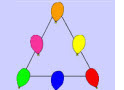# Math Logic Games

Do you know how to solve math logic games? Logic is very important when solving any types of math problems and even real-life scenarios. Sharpen your thinking skills by solving puzzles and games like the ones on this page.

Try one of these interesting and interactive online games!In this triangle logic game you are to place nine different numbers inside the six given baloons, so that the sum on every side of the triangle is equal to the same given number.In this magic square game you have to place nine given numbers inside the cells of a square, in order to obtain the same number, 15, when adding numbers vertically, horizontally, or diagonally.

Return from Math Logic Games to Math Play homepage.